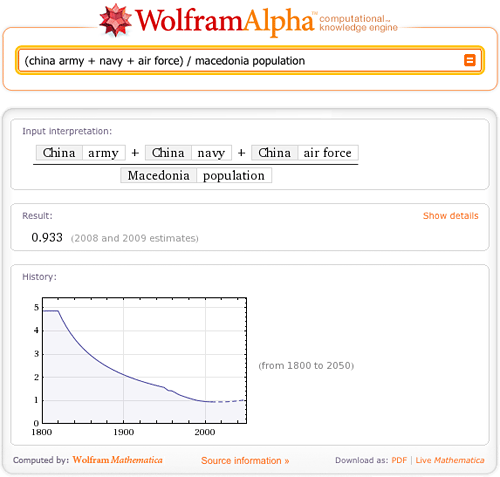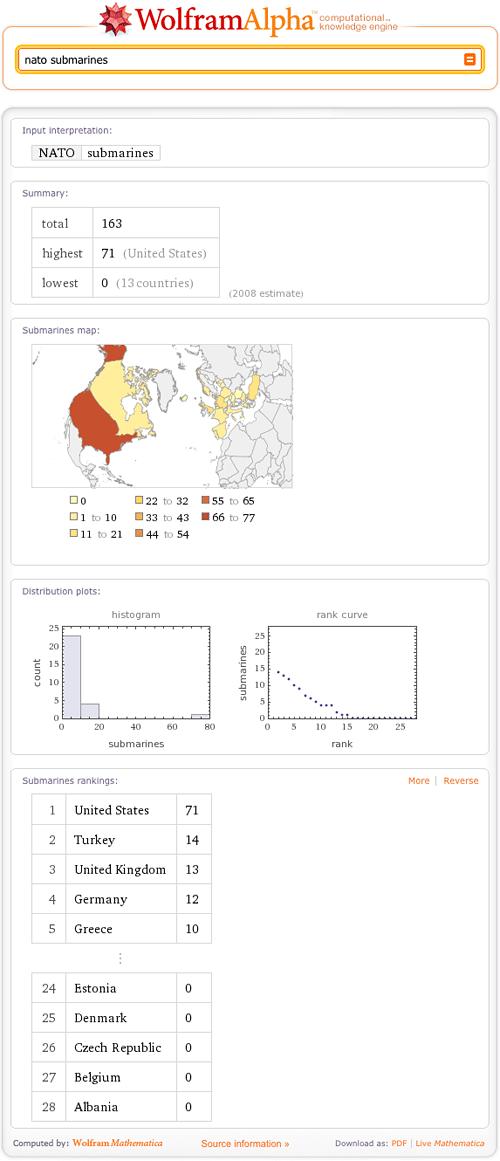# Wolfram|Alpha Builds an Arsenal of Military Data

June 1, 2010 —Comments Off

We’re in the midst of major enhancements to military data in Wolfram|Alpha, with newly added information on army, navy, and air force personnel for over 150 countries as well as statistics on many armaments, including stockpiles of nuclear warheads.

Let’s start with the big numbers. Type “army size of all countries” and you’ll see China, India, and the Korean Peninsula topping the list. China’s army alone includes 1.4 million soldiers and dwarfs the population of many smaller countries. The size of its combined army, navy, and air force is nearly equal to the entire population of Macedonia.There’s an abundance of data on armaments, around the world as well, including estimates on nuclear stockpiles of the nine countries known to have detonated nuclear weapons; according to the latest available estimates, Russia has the largest stockpile with 13,000 warheads. Also new in Wolfram|Alpha are figures on conventional weapons, including aircraft carriers, battle tanks, and fighter jets. Try comparing countries’ armaments, such as “tanks USA vs Russia”, or asking about the number of submarines in the NATO alliance.There’s more military information on the way in the coming months, including statistics on more types of armaments and international arms trade data. If this area of data is of interest to you and you would like to help expand this data, we invite you to consider joining the Wolfram|Alpha project as a volunteer data curator.

Hi, i have a problem in a graphic of a function.
f(x) = x^(1/3). See this graphics, is wrong!

Posted by John Guile June 1, 2010 at 2:36 pm

The graph shown of f (x) = x^(1/3) is actually correct. It displays not only the real values of the cubed root function, but also the complex values. Every real number has a unique real cube root, and every nonzero complex number has three distinct cube roots.

For example, the cubed root of 1 has the corresponding values 1 (which is the unique real cube root), -1/2 + 3^(1/3) I/2, and – 1/2 – 3^(1/3) I/2.

This graph in Wolfram|Alpha shows all three of these values where the red curve displays the imaginary parts and the blue curve displays the real parts.

Posted by The PR Team June 1, 2010 at 4:02 pm

What a most exciting adventure. Like a kid in a candy store. I will be visiting quite often.

Posted by John Cunningham June 1, 2010 at 8:39 pm

That’s gorgeous!

Posted by Mazinga June 3, 2010 at 1:52 am

great!!!!!!!!!!!! this site its my favorite!!!! =)

Posted by Francisco June 7, 2010 at 11:37 am

It is such a helpful resource which you are supplying and you’re giving it away for free. I honestly like discovering web sites that realize the value of supplying a useful resource for free. I really loved reading your post 🙂 Thank you

Posted by Antone Mulich June 23, 2010 at 7:37 pm

To find a root of the complex number a+ib, you find the arcotangent below 90 degrees of the complex ratio a/b, and then divide it by the root required. The cotangent of the result gives one of the say 3 roots of the complex ratio. For the other two roots you add on to the arcotangent 360 degrees and then 720 degrees. This procedure applies for all integer roots of complex numbers, so that there are always n nth roots of a complex number.

Posted by Peter L. Griffiths May 19, 2011 at 9:01 am

Further to my comment of 19 May 2011, the roots of imaginary numbers can be computed by letting a in the complex number a+ib equal 0 and substituting Cotes’s format cosu+isinu. With a=0 the angle u could be 90 degrees, so that cos90+isin90 will be 0+i. The doubling of 90 to 180 will give cos180+isin180 equals i squared which is -1. Likewise cos360+isin360 equals i to the power of 4 which is +1. This also applies to cos45+isin45 which is the square root of i. In this way we can arrive at the n nth roots of imaginary numbers, +1 and -1.

Posted by Peter L. Griffiths August 17, 2011 at 11:03 am

Hamilton’s quaternions equation i^2=j^2=k^2=ijk=-1 is incorrect in that -1 like all real imaginary and complex numbers cannot have more than two square roots, more than three cube roots, more than four fourth roots, more than five fifth roots etc. This means that k^2=-1 is wrong unless k equals either i or j.

Posted by Peter L. Griffiths March 4, 2012 at 11:57 am

Further to my comment of 4 March 2012, -1 cannot have more than two square roots, but -1 can have three cube roots which are cos60+isin60, cos180+isin180 which equals -1, and cos300+isin300. Hamilton seemed to have no understanding of these matters.

Posted by Peter L. Griffiths March 6, 2012 at 12:17 pm

Why do the roots of +1,-1,+i, and -i always add up to zero? The answer is that computing all these roots is a matter of going round the angles of a circle and finishing at the 360 degrees (=0 degrees) starting point. Incidentally if you have computed the roots of +i then the roots of -i are easily obtained by substituting -i for +i.

Posted by Peter L. Griffiths March 8, 2012 at 11:59 am